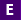#Not a Florida public school educator?

Access this resource
on CPALMS.com

### General Information

Subject(s): Mathematics
Grade Level(s): 7, 9, 10, 11, 12
Intended Audience: Educators, Students
Suggested Technology: Computer for Presenter, Computers for Students, Internet Connection, LCD Projector, Java Plugin
Instructional Time: 30 Minute(s)
Keywords: Plinko, Probability, Simulation, histogram, random, distribution, probability model
Instructional Component Type(s):
Instructional Design Framework(s): Demonstration, Learning Cycle (e.g., 5E)
Resource Collection: iCPALMS

## Aligned Standards

This vetted resource aligns to concepts or skills in these benchmarks.

## 1 Lesson Plan

Marble Mania In this lesson, "by flipping coins and pulling marbles out of a bag, students begin to develop a basic understanding of probabilities, how they are determined, and how the outcome of an experiment can be affected by the number of times it is conducted." (from Science NetLinks)

## Related Resources

Other vetted resources related to this resource.

## Lesson Plans

Marble Mania:

In this lesson, "by flipping coins and pulling marbles out of a bag, students begin to develop a basic understanding of probabilities, how they are determined, and how the outcome of an experiment can be affected by the number of times it is conducted." (from Science NetLinks)

Type: Lesson Plan

Who Would Have Figured? (Probability):

"Students discover what happens when a coin is tossed a few times versus when a coin is tossed many times. They discover the answer to "What is the probability of heads, and does it change as you toss the coin more times?" from Beacon Learning Center.

Type: Lesson Plan